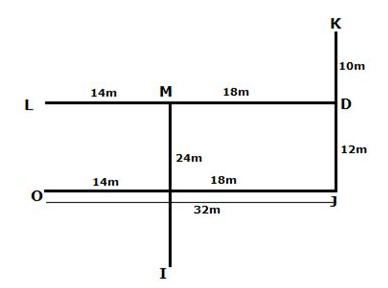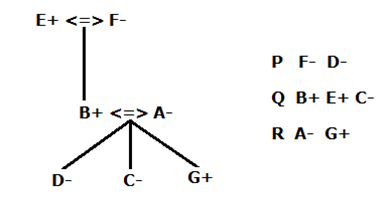# RRB Clerk Mains Reasoning (Day-11)

## Reasoning Materials for RRB Clerk Prelims:

Dear Aspirants, Our IBPS Guide team is providing new series of Reasoning Questions for RRB Clerk Mains 2020 so the aspirants can practice it on a daily basis. These questions are framed by our skilled experts after understanding your needs thoroughly. Aspirants can practice these new series questions daily to familiarize with the exact exam pattern and make your preparation effective.

Start Quiz

Input output

Direction (1-5): Study the following information to answer the given questions:

A number arrangement machine when given an input line of numbers rearranges them following a particular rule. The following is an illustration of input and re-arrangement.

Input: 57 18 25 88 96 34 19 48 77 66 29 54

Step I: 20 57 25 88 34 19 48 77 66 29 54 94

Step II: 21 20 57 25 34 48 77 66 29 54 94 86

Step III: 27 21 20 57 34 48 66 29 54 94 86 75

Step IV: 31 27 21 20 57 34 48 54 94 86 75 64

Step V: 36 31 27 21 20 48 54 94 86 75 64 55

Step VI: 50 36 31 27 21 20 94 86 75 64 55 52

And Step VI is the last step of the rearrangement of the above input.

As per the rules followed in the above steps, find out in each of the following questions the appropriate steps for the given input.

Input: 34 89 27 58 92 15 46 69 96 80

1) What is the sum of the number which is third from the right end and the number which is fourth from the left end in step 4?

A.130

B.119

C.107

D.97

E. None of these

2) In which of the following step “58 69 94” was found in the same order?

A. Step -1

B. Step -2

C. Step -3

D. Step -4

E. Step -5

3) What will be the resultant if the second number from the left end in step -2 is subtracted from the fifth number from the right end in step -3?

A.54

B.52

C.67

D.88

E. None of these

4) Which of the following number is third to the left of the number which is ninth from the left end in last step?

A.17

B.90

C.94

D.87

E. None of these

5) If the odd number is increased by 13 and even number is decreased by 15 in third step; then what is the product of lowest and fourth lowest number of the newly formed series?

A.872

B.972

C.652

D.986

E.882

Coded direction sense

Direction (6-7): Study the following information carefully and answer the questions below.

P \$ Q means P is north of Q

P & Q means P is south of Q

P @ Q means P is east of Q

P # Q means P is west of Q

P4* Q means P is at a distance of 4(meter) to Q

M14*@L, I24*&M18*#D10*&K, O32*#J12*&D

6) In which direction is point O with respect to the point I?

A.North-west

B.South-east

C.North

D.South-west

E.North-east

7) In which direction and distance is point K with respect to point J?

A.North-20

B.South-22

C.North-22

D.West-24

E.East-24

Blood relation

Direction (8-9): Study the following information carefully to answer the given questions.

A, B, C, D, E, F and G are seven members of a family belonging to three generations. There are two married couples from the first and second generations. They travel in different cars – P, Q and R so that no car has used more than three members and each car contains at least one female member. C neither travels with her mother nor belongs to the second generation. B travels with his father E in car Q. F travels only with her granddaughter D in car P. A travels with her only son in car R. B does not travel with his son in the same car.

8) How many female members are in the family?

A.1

B.2

C.4

D.3

E.None of these

9) In which car contain three members?

A.P

B.Q

C.R

D.Q or R

E. None of these

Critical reasoning

Direction (10): In the question below a statement is followed by two assumptions numbered I and II. An assumption is something supposed or taken for granted. You have to consider the statement and the following assumptions and then decide which of the assumptions is implicit in the statement.

10) Statement: The railway has maintained the best safety record in 2017-2018 fiscal in over five decades, according to official figures.

Assumptions:

I) The casualty figures also have gone down. In 2016-17, 607 people were injured or killed in train accidents, in 2017-2018, the number stood at 254.

II) Derailments have come down from 78 in 2016-2017 to 54 in 2017-2018, accidents at level crossings stood at 13 in 2017-2018 as compared to 30 the previous year.

A.Only assumption I is implicit

B.Only assumption II is implicit

C.Either assumption I or assumption II is implicit

D.Neither assumption I nor assumption II is implicit

E.Both assumption I and assumption II are implicit

Directions (1-5):

For step -1 lowest number is increased by 2 and it is arranged at left end and also highest number is decreased by 2 and it is arranged at right end

For step -2, Second lowest number is increased by 2 and it is arranged at left end and also Second highest number is decreased by 2 and it is arranged at right end and so on for the remaining steps.

Input: 34 89 27 58 92 15 46 69 96 80

Step I: 17 34 89 27 58 92 46 69 80 94

Step II: 29 17 34 89 58 46 69 80 94 90

Step III: 36 29 17 58 46 69 80 94 90 87

Step IV: 48 36 29 17 58 69 94 90 87 78

Step V: 60 48 36 29 17 94 90 87 78 67

Step 4 – 48 36 29 17 58 69 94 90 87 78

The sum of the number which is third from the right end and the number which is fourth from the left end in step 4 = 90+17 =107

In step -4 58 69 94 appeared in the same sequence

Second number from the left end in step -2 – 17

Fifth number from the right end in step -3 – 69

69-17 =52

Step -5 (last step) – 60 48 36 29 17 94 90 87 78 67

94- Third to the left of the number which is ninth from the left end in last step.

Odd number is increased by 13 and even number is decreased by 15 in third step – 36 29 17 58 46 69 80 94 90 87 becomes 21 42 30 43 31 82 65 79 75 100

Lowest number – 21

Fourth lowest number – 42

21*42 = 882

Directions (6-7):Directions (8-9):